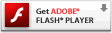# Interactive Generative Flash Art Series – 00 – Line Width

After seeing the source code in the original experiment, I found myself wanting to play with the code. I saw many elements of the lines and actions that could be interesting to explore. This is the first in a series, of experiments I’ll post starting from this code and pushing different things each time. They’ll start slow and simple, like this one. All I’m doing is adding a few lines of code. The first thing I wanted to see from the original was a more gradual step in the width of the line. Here are a few screenshots from this iteration:

### Play here

Please visit the blog article to view this interactive flash content. Flash plug-in required:### Source Code

[cc lang=”actionscript”]
var ball:Sprite = new Sprite();
ball.graphics.beginFill(0x333333, 1);
ball.graphics.drawCircle(0, 0, 30);
ball.graphics.endFill();

var div:Number = .1;
var ax:Number = 0;
var ay:Number = 0;

var line_max_width:Number = 30;
var line_min_width:Number = 0;
var line_width:Number = Math.random() * line_max_width;
var line_width_velocity:Number = 0;
var dampen:Number = 0.95;

function loop () {
ball.x -= ax = (ax + (ball.x – mouseX) * div) * .9;
ball.y -= ay = (ay + (ball.y – mouseY) * div) * .9;

line_width_velocity += Math.random() * 4 – 2;
line_width += line_width_velocity;
line_width_velocity *= dampen;
if(line_width > line_max_width) { line_width = line_max_width; }
if (line_width < line_min_width) { line_width = line_min_width; }
this.graphics.lineStyle(line_width, 0, 1);
this.graphics.lineTo(ball.x, ball.y);
}

setInterval(loop, 1000/30);
[/cc]

### Method Explained

If you need a little walk through on my method to achieve this, all I did was create a variable to hold my line width, and the line width velocity (or rate of change). Every frame the line velocity grows or shrinks randomly and is applied to the line width variable. I apply a dampen to the velocity so it doesn’t get too out of hand and then set some limits to the line width. Pretty simple and it applies the same method of applying velocity to an object to make it move, but this velocity is being applied to a property of the object (width) that may not be as obvious as position. This still gives an effect of random width to the lines, but they are randomly widening and thinning, rather than just having random widths. It gives it a more fluid appearance. Any other ways you would use to achieve the same effect?

1.Don Booth says: